Cis/Trans stereoisomers in 2 or 3 Dimensions

If the atomic coordinates are specified, then the molecule has nonzero spatial dimension. In this case the double bond stereo information is calculated from the double bond and the reference frame atom coordinates. There are two exceptions when coordinates are not used and CIS|TRANS value is returned:

1. CIS|TRANS flag is set for the double bond which is depicted as crossed double bond.2. One ligand of the double bond has wiggly (UP|DOWN) bond type.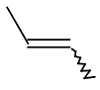Setting cis/trans information in 2 or 3 Dimensions

The only way to change the double stereo information from CIS to TRANS in 2 or 3D is to change the atomic coordinates of the corresponding ligands. This can be achieved through setXYZ(double x, double y, double z) or setXY(double x, double y) functions of MolAtom class. Be aware that modifying the coordinates directly may cause overlapping atoms and bonds. It is more convenient to convert the molecule to 0D, change stereo information in the 0D molecule and clean it to 2D or 3D.

Code example: Changing double bond type through 0 dimension

`molecule.setDim(``0``); `

`// reference frame `
`MolAtom a1 = molecule.getAtom(``0``); `
`MolAtom a4 = molecule.getAtom(``4``);`

`// set CIS value to the reference frame `
`MolBond b = molecule.getBond(``1``); `
`b.setStereo2Flags(a1, a4, StereoConstants.CIS); `

`// clean to 2D `
`molecule.clean(``2``, ``null``); `
`To change the actual value to CIS|TRANS, there are two possibilities: either set CIS|TRANS flag for the double bond or set one bond between the double bond and a ligand to wiggly.`

Code example: set the double bond to CIS|TRANS via changing the double bond flag

`int`` CISTRANS = StereoConstants.CIS | StereoConstants.TRANS;`
`// get the double bond`
`MolBond b = molecule.getBond(``2``);`
`// change flag`
`b.setFlags(CISTRANS, StereoConstants.CTUMASK); `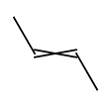Code example: set double bond to CIS|TRANS using wiggly bond:

`// get the double bond `
`MolBond b = molecule.getBond(``2``); `

`// get a single bond connected to one endpoint `
`// of the double bond`
`MolAtom a1 = b.getAtom1(); `
`MolBond s = (a1.getBond(``0``) == b) ? a1.getBond(``1``) : a1.getBond(``0``);`
`// change single bond to WAVY`
`s.setFlags(MolBond.WAVY, STEREO1_MASK);`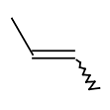Getting cis/trans information in 2 or 3 Dimensions

There are two low level functions in MolBond class to calculate double bond stereo information from the coordinates in 2D or 3D. None of them checks if the bond in question is double bond or not.

• calcStereo2() calculate the stereo information for the default reference frame.

• calcStereo2(MolAtom atom1, MolAtom atom4) calculates the stereo information for the given reference frame. Note: it is not checked if atom1 and atom4 are bound to the bond.

As in case of the 0D molecules there are four methods to get the stereo information with reference frame given:

1. getStereo2(MolAtom a1, int i2, int i3, MolAtom a4)

2. getStereo2(int i1, int i2, int i3, int i4)

3. getStereo2(MolBond b, MolAtom a1, MolAtom a4)

4. getStereo2(MolBond b, MolAtom a1, MolAtom a4, boolean grcheck)

All of them are functions of the MoleculeGraph class. The difference between them is how the reference frame is specified (by the node or by the node index) and the last method can check atom equivalences too.

It is important to note that CIS or TRANS result can be obtained if and only if there is no contradictory information in the coordinates. This means that two ligands at one end of the double bond on the same side are not allowed. Moreover, if a ligand is collinear with the double bond CIS|TRANS value is returned: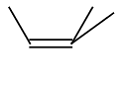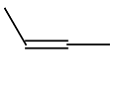Stereo value: 0 - if graph invariances are checked on the ligands CIS | TRANS - if graph invariances are not checked on the ligands Stereo value: CIS | TRANS

Code example: without atom equivalence check:

`MolBond b = molecule.getBond(``2``); `
`// reference frame`
`MolAtom a1 = molecule.getAtom(``0``); `
`MolAtom a4 = molecule.getAtom(``4``); `
`int`` s = molecule.getStereo2(b, a1, a4);`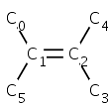s = CIS

Code example: atom equivalence check

`MolBond b = molecule.getBond(``2``);`
`// reference frame`
`MolAtom a1 = molecule.getAtom(``0``); `
`MolAtom a4 = molecule.getAtom(``4``);`
`int`` s = molecule.getStereo2(b, a1, a4, ``true``);`s = 0